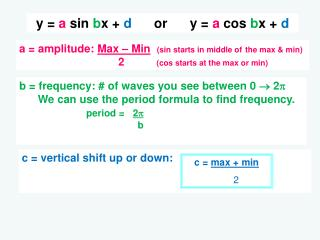DownloadDownload Presentationy = a sin b x + d or y = a cos b x + d

# y = a sin b x + d or y = a cos b x + d

Télécharger la présentation## y = a sin b x + d or y = a cos b x + d

- - - - - - - - - - - - - - - - - - - - - - - - - - - E N D - - - - - - - - - - - - - - - - - - - - - - - - - - -
##### Presentation Transcript

1. y = asinbx + dory = acosbx + d a = amplitude: Max – Min(sin starts in middle ofthe max & min) 2 (cos starts at the max or min) b = frequency: # of wavesyou see between 0  2 We can use the period formula to find frequency. period = 2 b c = vertical shift up or down: c = max + min 2

2. #1 Given the graph, find the function. y = sinx + 1 sin y = _________ x + 1 1 1 AMPLITUDE = Max – Min 2 SHIFT = Max + Min 2 FREQUENCY b # of curves from 0 to 2 Period = 2 b = 2 – 0 2 = 1 = 2 + 0 2 = 1 b= 1

3. #2 y = cos 2x + 3 cos y = _________ x + 1 2 3 AMPLITUDE = Max – Min 2 SHIFT = Max + Min 2 FREQUENCY # of curves from 0 to 2 Period = 2 b = 4 – 2 2 = 1 = 4 + 2 2 = 3 b= 2

4. #3 y = - 3sin8x sin y = _________ x + -3 8 0 AMPLITUDE = Max – Min 2 SHIFT = Max + Min 2 FREQUENCY # of curves from 0 to 2 P = 2 b = 3 – -3 2 = 3 UPSIDE DOWN = 3+ -3 2 = 0 P =/4  =2 4 b b = 8

5. #4 y= - 2cos4x + 4 y = _________ x + cos 4 -2 4 AMPLITUDE = Max – Min 2 SHIFT = Max + Min 2 FREQUENCY # of curves from 0 to 2 Period = 2 b = 6 – 2 2 = 2 UPSIDE DOWN = 6+ 2 2 = 4 P =/2 2 =  b 2 b = 4

6. #5 y = 3sin(x – π/2 ) + 1 sin y = _________ (x - ) + 3 π/2 1 1 AMPLITUDE = Max – Min 2 Vertical SHIFT = Max + Min = 4 + -2 =1 2 2 FREQUENCY # of curves from 0 to 2 Period = 2 b = 4 – -2 2 = 3 P = 2 2 = 2 b b = 1 Phase SHIFT right π/2

7. #6 y= 4cos4(x -π/4) 4 cos 4 y = _______ (x - ) + π/4 0 AMPLITUDE = Max – Min 2 Vertical SHIFT = Max + Min = 4 + -4 = 0 FREQUENCY # of curves from 0 to 2 Period = 2 b 2 2 = 4– -4 2 = 4 Phase SHIFT right π/4 P = π/2 2 = π b 2 b = 4

8. Translating Trig Curves Remember a translation SLIDES all points on a curve the same distance and direction. DO NOW: Sketch f(x) = 2 cos x for one cycle. a. Sketch f(x) = 2 cos x + 3 b. Sketch f(x) = 2 cos x - 4